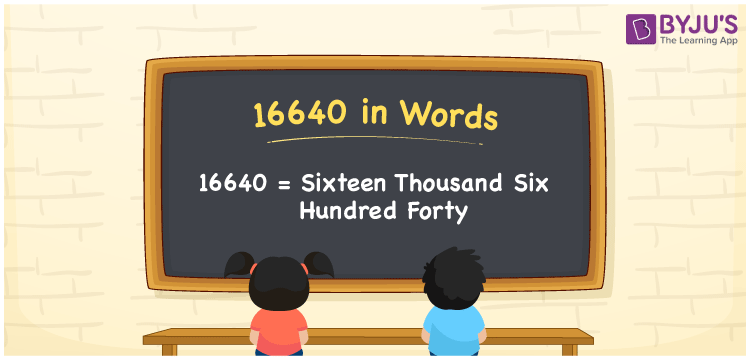# 16640 in Words

We can write the number 16640 in words as Sixteen thousand six hundred forty. If you purchased a drone camera that costs Rs. 16640, you can say, “I purchased a drone camera worth Sixteen thousand six hundred forty rupees”. Here, Sixteen thousand six hundred forty is the number name or word form for 16640. Also, we know that 16640 is a cardinal number since it describes a specific quantity.

 16640 in words Sixteen thousand six hundred forty Sixteen thousand six hundred forty in Numbers 16640

## 16640 in English Words

Generally, we use the English alphabet to express numbers in words. Thus, we spell 16640 in English words as “Sixteen thousand six hundred forty”.## How to Write 16640 in Words?

The number 16640 has five digits so, let’s make a place value chart in such a way that we can define place values for all the digits.

 Ten thousands Thousands Hundreds Tens Ones 1 6 6 4 0

Here, ones = 0, tens = 4, hundreds = 6, thousands = 6, ten thousands = 1

The above numbers can be expanded as follows.

1 × Ten thousand + 6 × Thousand + 6 × Hundred + 4 × Ten + 0 × One

= 1 × 10000 + 6 × 1000 + 6 × 100 + 4 × 10 + 0 × 1

= 10000 + 6000 + 600 + 40

= Ten Thousand + Six thousand + Six hundred + Forty

= Sixteen thousand six hundred forty

Hence, 16640 in words = Sixteen thousand six hundred forty.

16640 is a natural number that is the successor of 16639 and the predecessor of 16641.

16640 in words – Sixteen thousand six hundred forty

Is 16640 an odd number? – No

Is 16640 an even number? – Yes

Is 16640 a perfect square number? – No

Is 16640 a perfect cube number? – No

Is 16640 a prime number? – No

Is 16640 a composite number? – Yes

## Frequently Asked Questions on 16640 in Words

Q1

### How to write 16640 in words?

We can write the number 16640 in words as Sixteen thousand six hundred forty.
Q2

### How do you write Rs. 16640 in words on a cheque?

On a cheque, we generally write Rs. 16640 in words as “Sixteen thousand six hundred forty rupees only”.
Q3

### Express 166.40 in words.

We can write 166.40 in words as: 166.40 = One hundred sixty-six and four-tenths (or) 166.40 = One hundred sixty-six point four zero